# Separating Stimulus, Saccade and Buttonpress

This example has been used in the unfold toolbox paper Ehinger & Dimigen 2019, peerJ. For more details on the experiment please have a look there!
init_unfold
Starting unfold toolbox. Adding subfolders and other toolboxes to path... Done.

# Introduction

Subjects are exploring a face stimulus. Using an eyetracker and the EEG-Eye toolbox we add markers of saccade onsets, stimulus onsets and button presses into our EEG-Data. The data were cleaned for eye-movement related artefacts using ICA.
In this tutorial we try to disentangle stimulus related ERPs, buttonpress ERPs and (micro)saccade related ERPs.
Find the data at: https://osf.io/wbz7x/
% EEG = pop_loadset('C:\Users\behinger\Dropbox\deconv_olaf\workshop\data\facestudy_integrated.set')
EEG = struct with fields:
setname: 'face_saccades_opendata' filename: 'face_saccades_opendata_fig10.set' filepath: 'C:\Users\behinger\Downloads' subject: '' group: '' condition: '' session: [] comments: 'Data to re-create Figure 10 in Ehinger & Dimigen, 2018, BioRxiv. Please do not use this data for other purposes without asking first (olaf.dimigen@hu-berlin.de)' nbchan: 40 trials: 1 pnts: 2070402 srate: 500 xmin: 0 xmax: 4.1408e+03 times: [1×2070402 double] data: [40×2070402 single] icaact: [] icawinv: [] icasphere: [] icaweights: [] icachansind: [] chanlocs: [1×40 struct] urchanlocs: [] chaninfo: [1×1 struct] ref: 'averef' event: [1×7661 struct] urevent: [1×7661 struct] eventdescription: {'' '' '' '' '' '' '' '' '' '' '' '' '' ''} epoch: [] epochdescription: {} reject: [1×1 struct] stats: [1×1 struct] specdata: [] specicaact: [] splinefile: '' icasplinefile: '' dipfit: [] history: '↵EEG.setname='MSAFF_01';↵EEG = eeg_checkset( EEG );↵EEG = eeg_checkset( EEG );↵EEG.etc.eeglabvers = '14.1.2'; % this tracks which version of EEGLAB is being used, you may ignore it↵EEG = pop_loadset('filename','face_saccades_opendata.set','filepath','M:\\Dropbox\\shared folders_main\\deconv\\figures\\toolbox_paper\\redo_figure10_to_match_opendata\\');↵EEG = eeg_checkset( EEG );↵pop_eegplot( EEG, 1, 1, 1);↵EEG = pop_select( EEG,'nochannel',{'L-Dia-X-(px)' 'L-Dia-Y-(px)' 'R-Dia-X-(px)' 'R-Dia-Y-(px)'});↵EEG = eeg_checkset( EEG );↵EEG = pop_saveset( EEG, 'filename','face_saccades_opendata_fig10.set','filepath','M:\\Dropbox\\shared folders_main\\deconv\\figures\\toolbox_paper\\redo_figure10_to_match_opendata\\');↵EEG = eeg_checkset( EEG );↵EEG = pop_select( EEG,'nochannel',{'L-GAZE-X-(px)' 'L-GAZE-Y-(px)' 'R-GAZE-X-(px)' 'R-GAZE-Y-(px)'});↵EEG = eeg_checkset( EEG );↵EEG.etc.eeglabvers = '14.1.1'; % this tracks which version of EEGLAB is being used, you may ignore it' saved: 'justloaded' etc: [1×1 struct] datfile: []

# Running the toolbox

cfgDesign = [];
cfgDesign.eventtypes = {'saccade','stimonset','buttonpress'}; % we model the saccadeonset, stimulusonset and buttonpress
cfgDesign.formula = {'y ~ 1 + spl(sac_amplitude,5)','y~1','y~1'}; % For splines see the spline tutorial
EEG = uf_designmat(EEG,cfgDesign);
Multiple events with separate model-formula detected Modeling 2871 event(s) of [saccade] using formula: y~1 The spline that got removed due to collinearity in the basis set (as intended) for the effect sac_amplitude has its peak at 0.410368 This does not mean that the event-intercept represents this value! Modeling 479 event(s) of [stimonset] using formula: y~1 Modeling 479 event(s) of [buttonpress] using formula: y~1
Timexpand everything:
cfgTimeexpand = [];
cfgTimeexpand.timelimits = [-0.8 1];
EEG = uf_timeexpandDesignmat(EEG,cfgTimeexpand);
uf_timeexpandDesignmat(): Timeexpanding the designmatrix... ...done
Fit the GLM (solve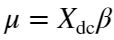for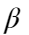)
EEG = uf_glmfit(EEG,'channel',32);
uf_glmfit(): Fitting deconvolution model...solving the equation system solving electrode 32 (of 1 electrodes in total)... 70 iterations, took 3.2s LMfit finished

# Massive-Univariate Model (without deconvolution)

it is nice to compare deconvolution results with the results of a normal massive-univariate regression (this regression will be identical to a simple ERP for stimulus & response). To do so, we need to epoch our data and fit a linear model for each timepoint/electrode separately.
EEG_epoch = uf_epoch(EEG,'timelimits',cfgTimeexpand.timelimits);
pop_epoch():3829 epochs selected Epoching... pop_epoch():3829 epochs generated eeg_checkset: found empty values for field 'timeSinceLastStimulus' filling with values of other events in the same epochs Event resorted by increasing latencies. pop_epoch(): checking epochs for data discontinuity Recalculating the EEG_epoch field using eeg_checkset, might take some time Event resorted by increasing latencies.
EEG_epoch = uf_glmfit_nodc(EEG_epoch);
% Unfold does not overwrite the deconv-betas, but can keep both at the same time

# Combine results & plot

ufresult = uf_condense(EEG_epoch);
% ufresult.beta => deconvolved
% ufresult.beta_nodc => Massive-Univariate
% evaluate the spline at some points of the continuous saccade-amplitude spectrum
ufresult = uf_predictContinuous(ufresult,'predictAt',{{'sac_amplitude',0.75:0.5:3.5}})
ufresult = struct with fields:
unfold: [1×1 struct] times: [1×900 double] chanlocs: [1×40 struct] param: [1×9 struct] beta_nodc: [40×900×9 double] beta: [40×900×9 double]
% We always only plot one predictor. The quesiton is, at what value of the other predictors, should we look at it?
% Here we look at one predictor when all other predictors are at their respective mean value.
% Plot them
uf_plotParam(ufresult,'channel',32,'plotSeparate','event');
plotting all parameters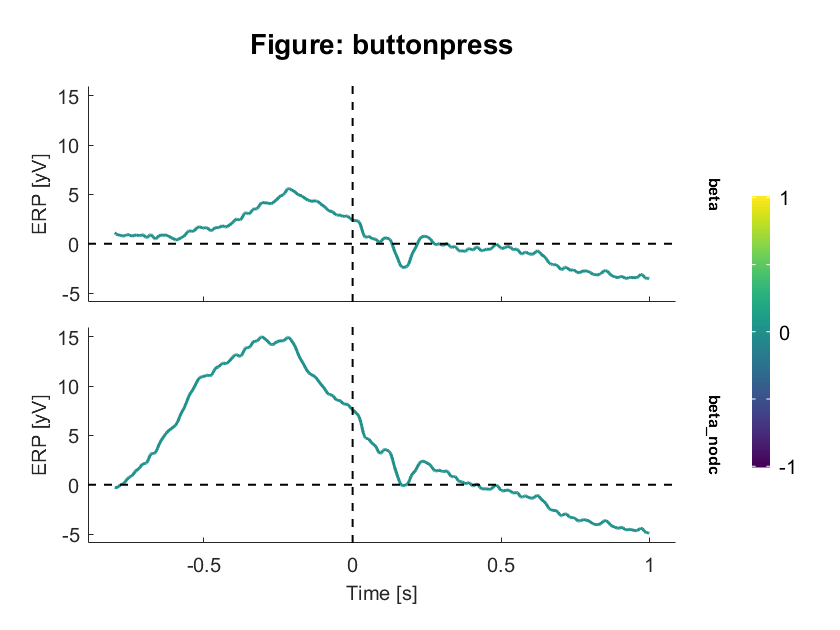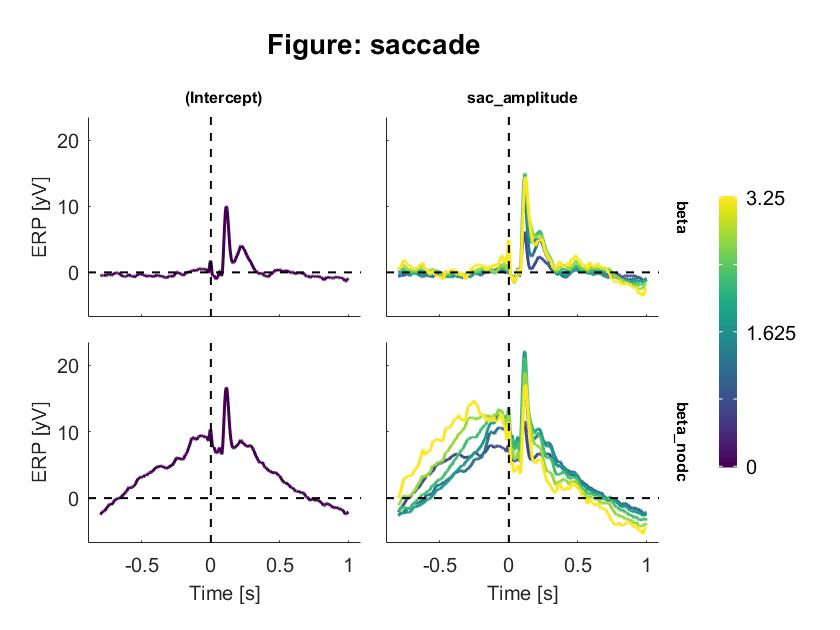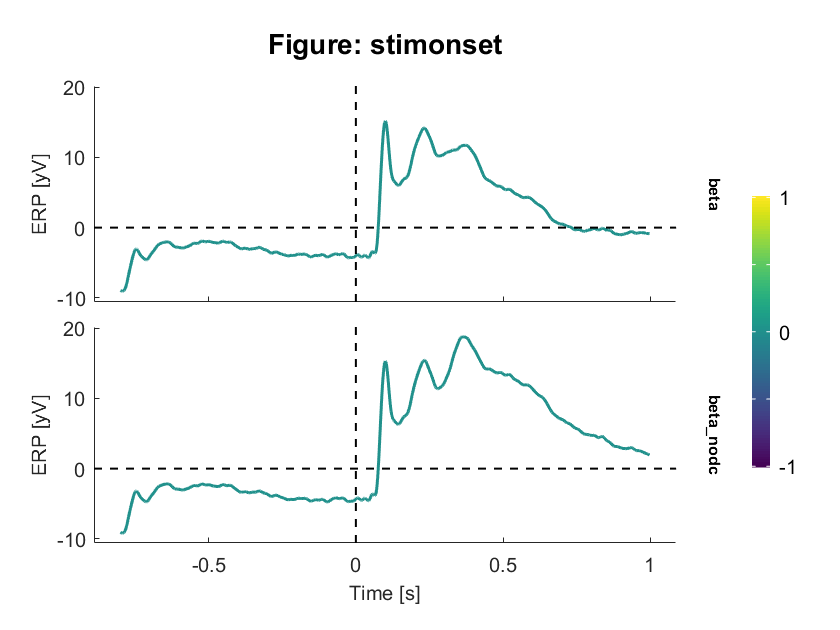It is very apparent that much of the button-press related activity visible in the "no-deconvolution" (classical ERP) condition is removed by the deconvolution. The same holds true for the saccade effect. The stimulus effect is also different, but only at later time points (~250ms).

## ERPImages

An erpimage for stimulus onset "raw"
uf_erpimage(EEG_epoch,'channel',32,...
'alignto','stimonset',...
'sort_time',[0.2 0.8],...
'type','raw',...
'timelimits',[-.2 1])
Aligning erpimage to event stimonset, 480 epochs found Sorting erpimage from event saccade forwards by field latency Plotting input data as 480 epochs of 900 frames sampled at 500.0 Hz. Removing 5 trials with NaN sortvar values. Sorting data on input sortvar. 92.42% of the trials (i.e., 439 out of 475) have the same sortvar value as at least one other trial. Distribution of number ties per unique value of sortvar: Min: 0, 25th ptile: 0, Median: 2, 75th ptile: 5, Max: 17 Smoothing the sorted epochs with a 10-epoch moving window. and a decimation factor of 1 Using the specified caxis range of [-22.482,22.482]. Data will be plotted between -800 and 998 ms.
Output data will be 900 frames by 466 smoothed trials. Outtrials: 6.00 to 471.00
Overplotting sorted sortvar on data.
Done.
An erpimage for stimulus onset "modelled"
uf_erpimage(EEG,'channel',32,...
'alignto','stimonset',... # which event is at "0"
'sort_alignto','saccade',... # which event should it be sorted by?
'sort_time',[0.2 0.8],... # in which time to look for the sorting event
'type','modelled',...# in this case use beta_dc (=> yhat)